## Graphing and Writing Equations of Linear Functions

### Learning Outcomes

• Graph linear functions by plotting points, using the slope and y-intercept, and using transformations.
• Write the equation of a linear function given its graph.
• Match linear functions with their graphs.
• Find the x-intercept of a function given its equation.
• Find the equations of vertical and horizontal lines.
• Determine whether lines are parallel or perpendicular given their equations.
• Find equations of lines that are parallel or perpendicular to a given line.
• Graph an absolute value function.
• Find the intercepts of an absolute value function.

We can now describe a variety of characteristics that explain the behavior of linear functions. We will use this information to analyze a graphed line and write an equation based on its observable properties. From evaluating the graph, what can you determine about this linear function?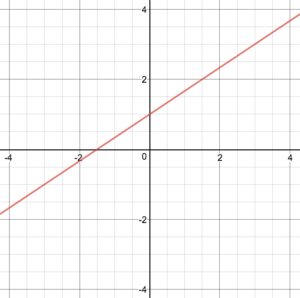• initial value (y-intercept)?
• one or two points?
• slope?
• increasing or decreasing?
• vertical or horizontal?

In this section, you will practice writing linear function equations using the information you’ve gathered. We will also practice graphing linear functions using different methods and predict how the graphs of linear functions will change when parts of the equation are altered.

## Graphing Linear Functions

We previously saw that that the graph of a linear function is a straight line. We were also able to see the points of the function as well as the initial value from a graph.

There are three basic methods of graphing linear functions. The first is by plotting points and then drawing a line through the points. The second is by using the y-intercept and slope. The third is applying transformations to the identity function $f\left(x\right)=x$.

### Graphing a Function by Plotting Points

To find points of a function, we can choose input values, evaluate the function at these input values, and calculate output values. The input values and corresponding output values form coordinate pairs. We then plot the coordinate pairs on a grid. In general we should evaluate the function at a minimum of two inputs in order to find at least two points on the graph of the function. For example, given the function $f\left(x\right)=2x$, we might use the input values 1 and 2. Evaluating the function for an input value of 1 yields an output value of 2 which is represented by the point (1, 2). Evaluating the function for an input value of 2 yields an output value of 4 which is represented by the point (2, 4). Choosing three points is often advisable because if all three points do not fall on the same line, we know we made an error.

### How To: Given a linear function, graph by plotting points.

1. Choose a minimum of two input values.
2. Evaluate the function at each input value.
3. Use the resulting output values to identify coordinate pairs.
4. Plot the coordinate pairs on a grid.
5. Draw a line through the points.

### Example: Graphing by Plotting Points

Graph $f\left(x\right)=-\frac{2}{3}x+5$ by plotting points.

### Try It

Graph $f\left(x\right)=-\frac{3}{4}x+6$ by plotting points.

### Graphing a Linear Function Using y-intercept and Slope

Another way to graph linear functions is by using specific characteristics of the function rather than plotting points. The first characteristic is its y-intercept which is the point at which the input value is zero. To find the y-intercept, we can set $x=0$ in the equation.

The other characteristic of the linear function is its slope, m, which is a measure of its steepness. Recall that the slope is the rate of change of the function. The slope of a linear function is equal to the ratio of the change in outputs to the change in inputs. Another way to think about the slope is by dividing the vertical difference, or rise, between any two points by the horizontal difference, or run. The slope of a linear function will be the same between any two points. We encountered both the y-intercept and the slope in Linear Functions.

Let’s consider the following function.

$f\left(x\right)=\frac{1}{2}x+1$

The slope is $\frac{1}{2}$. Because the slope is positive, we know the graph will slant upward from left to right. The y-intercept is the point on the graph when = 0. The graph crosses the y-axis at (0, 1). Now we know the slope and the y-intercept. We can begin graphing by plotting the point (0, 1) We know that the slope is rise over run, $m=\frac{\text{rise}}{\text{run}}$. From our example, we have $m=\frac{1}{2}$, which means that the rise is 1 and the run is 2. Starting from our y-intercept (0, 1), we can rise 1 and then run 2 or run 2 and then rise 1. We repeat until we have multiple points, and then we draw a line through the points as shown below.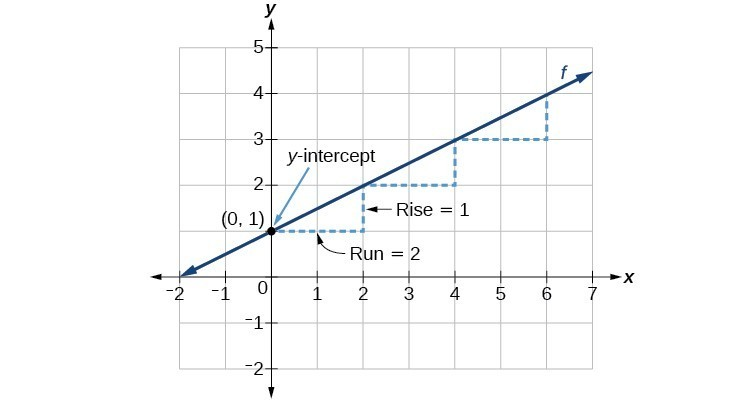### A General Note: Graphical Interpretation of a Linear Function

In the equation $f\left(x\right)=mx+b$

• b is the y-intercept of the graph and indicates the point (0, b) at which the graph crosses the y-axis.
• m is the slope of the line and indicates the vertical displacement (rise) and horizontal displacement (run) between each successive pair of points. Recall the formula for the slope:

$m=\frac{\text{change in output (rise)}}{\text{change in input (run)}}=\frac{\Delta y}{\Delta x}=\frac{{y}_{2}-{y}_{1}}{{x}_{2}-{x}_{1}}$

### Q & A

Do all linear functions have y-intercepts?

Yes. All linear functions cross the y-axis and therefore have y-intercepts. (Note: A vertical line parallel to the y-axis does not have a y-intercept. Keep in mind that a vertical line is the only line that is not a function.)

### How To: Given the equation for a linear function, graph the function using the y-intercept and slope.

1. Evaluate the function at an input value of zero to find the y-intercept.
2. Identify the slope.
3. Plot the point represented by the y-intercept.
4. Use $\frac{\text{rise}}{\text{run}}$ to determine at least two more points on the line.
5. Draw a line which passes through the points.

### Example: Graphing by Using the y-intercept and Slope

Graph $f\left(x\right)=-\frac{2}{3}x+5$ using the y-intercept and slope.

### Try It

Find a point on the graph we drew in the previous example: Graphing by Using the y-intercept and Slope that has a negative x-value.

### Graphing a Linear Function Using Transformations

Another option for graphing is to use transformations on the identity function $f\left(x\right)=x$. A function may be transformed by a shift up, down, left, or right. A function may also be transformed using a reflection, stretch, or compression.

### Vertical Stretch or Compression

In the equation $f\left(x\right)=mx$, the m is acting as the vertical stretch or compression of the identity function. When m is negative, there is also a vertical reflection of the graph. Notice that multiplying the equation $f\left(x\right)=x$ by m stretches the graph of f by a factor of m units if > 1 and compresses the graph of f by a factor of m units if 0 < < 1. This means the larger the absolute value of m, the steeper the slope.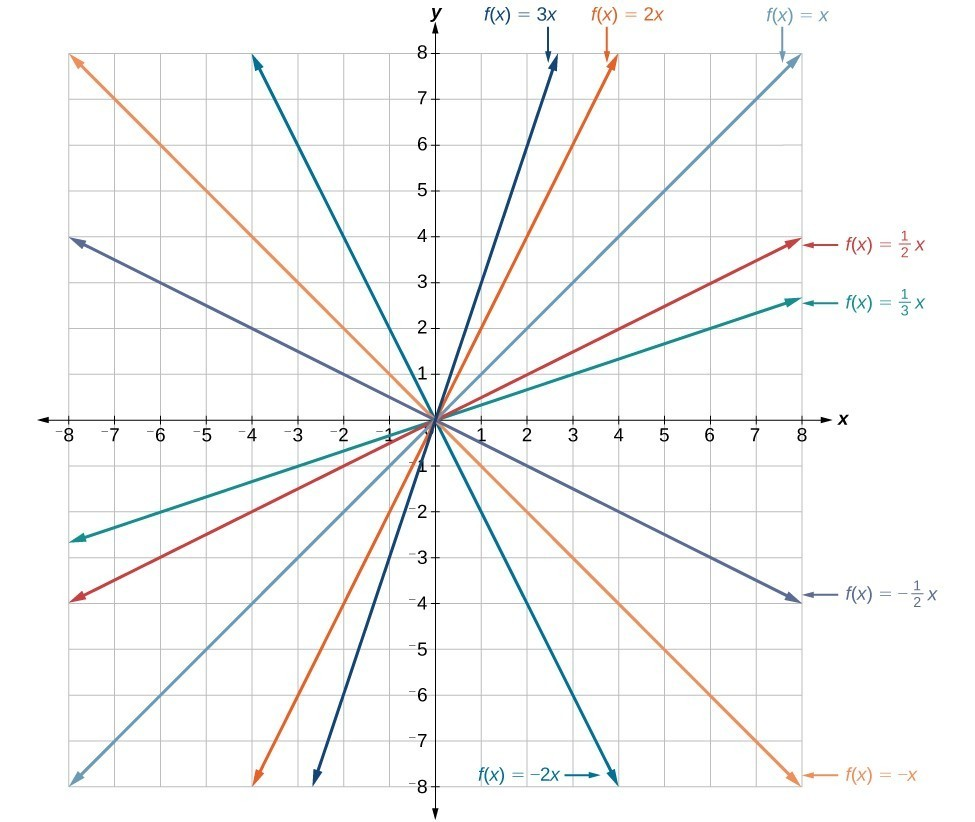Vertical stretches and compressions and reflections on the function $f\left(x\right)=x$.

### Vertical Shift

In $f\left(x\right)=mx+b$, the b acts as the vertical shift, moving the graph up and down without affecting the slope of the line. Notice that adding a value of b to the equation of $f\left(x\right)=x$ shifts the graph of f a total of b units up if b is positive and |b| units down if b is negative.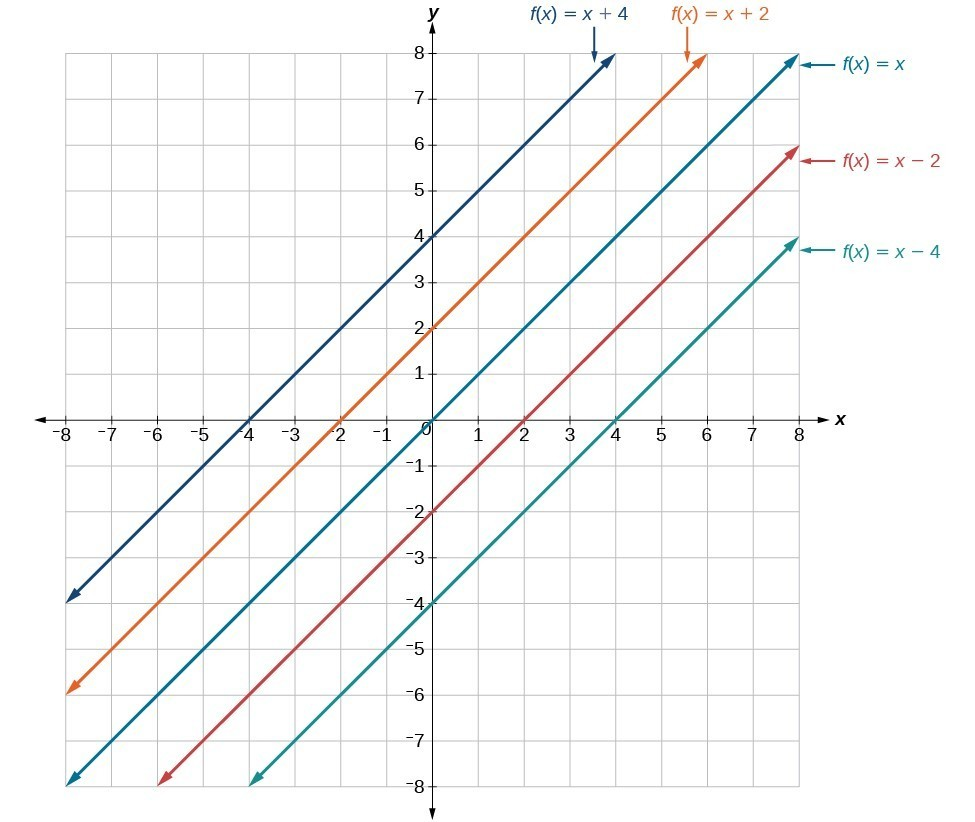This graph illustrates vertical shifts of the function $f\left(x\right)=x$.

Using vertical stretches or compressions along with vertical shifts is another way to look at identifying different types of linear functions. Although this may not be the easiest way to graph this type of function, it is still important to practice each method.

### How To: Given the equation of a linear function, use transformations to graph the linear function in the form $f\left(x\right)=mx+b$.

1. Graph $f\left(x\right)=x$.
2. Vertically stretch or compress the graph by a factor m.
3. Shift the graph up or down b units.

### Example: Graphing by Using Transformations

Graph $f\left(x\right)=\frac{1}{2}x - 3$ using transformations.

### Try It

Graph $f\left(x\right)=4+2x$, using transformations.

### Q & A

In Example: Graphing by Using Transformations, could we have sketched the graph by reversing the order of the transformations?

No. The order of the transformations follows the order of operations. When the function is evaluated at a given input, the corresponding output is calculated by following the order of operations. This is why we performed the compression first. For example, following order of operations, let the input be 2.

$\begin{array}{l}f\text{(2)}=\frac{\text{1}}{\text{2}}\text{(2)}-\text{3}\hfill \\ =\text{1}-\text{3}\hfill \\ =-\text{2}\hfill \end{array}$

## Writing Equations of Linear Functions

We previously wrote the equation for a linear function from a graph. Now we can extend what we know about graphing linear functions to analyze graphs a little more closely. Begin by taking a look at the graph below. We can see right away that the graph crosses the y-axis at the point (0, 4), so this is the y-intercept.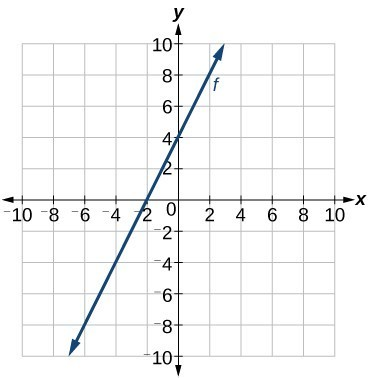Then we can calculate the slope by finding the rise and run. We can choose any two points, but let’s look at the point (–2, 0). To get from this point to the y-intercept, we must move up 4 units (rise) and to the right 2 units (run). So the slope must be:

$m=\frac{\text{rise}}{\text{run}}=\frac{4}{2}=2$

Substituting the slope and y-intercept into slope-intercept form of a line gives:

$y=2x+4$

### How To: Given THE graph of A linear function, find the equation to describe the function.

1. Identify the y-intercept from the graph.
2. Choose two points to determine the slope.
3. Substitute the y-intercept and slope into slope-intercept form of a line.

### Example: Matching Linear Functions to Their Graphs

Match each equation of a linear function with one of the lines in the graph below.

1. $f\left(x\right)=2x+3$
2. $g\left(x\right)=2x - 3$
3. $h\left(x\right)=-2x+3$
4. $j\left(x\right)=\frac{1}{2}x+3$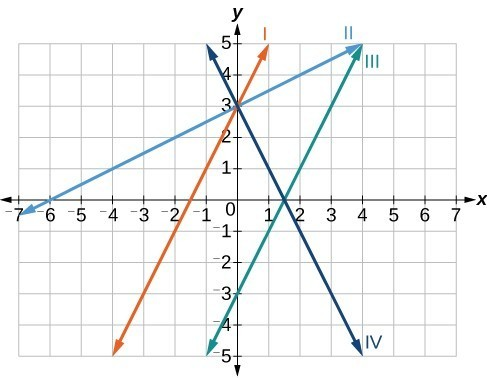### Finding the x-intercept of a Line

So far we have been finding the y-intercepts of functions: the point at which the graph of a function crosses the y-axis. A function may also have an x-intercept, which is the x-coordinate of the point where the graph of a function crosses the x-axis. In other words, it is the input value when the output value is zero.

To find the x-intercept, set the function f(x) equal to zero and solve for the value of x. For example, consider the function shown:

$f\left(x\right)=3x - 6$

Set the function equal to 0 and solve for x.

$\begin{array}{l}0=3x - 6\hfill \\ 6=3x\hfill \\ 2=x\hfill \\ x=2\hfill \end{array}$

The graph of the function crosses the x-axis at the point (2, 0).

### Q & A

Do all linear functions have x-intercepts?

No. However, linear functions of the form = c, where c is a nonzero real number are the only examples of linear functions with no x-intercept. For example, = 5 is a horizontal line 5 units above the x-axis. This function has no x-intercepts.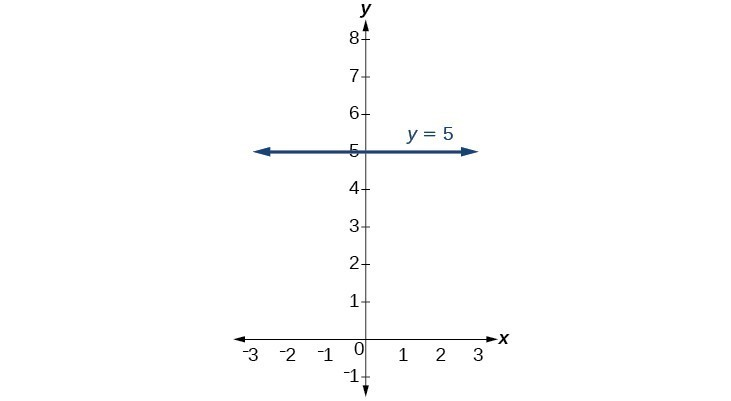### A General Note: x-intercept

The x-intercept of a function is the value of x where f(x) = 0. It can be found by solving the equation 0 = mx + b.

### Example: Finding an x-intercept

Find the x-intercept of $f\left(x\right)=\frac{1}{2}x - 3$.

### Try It

Find the x-intercept of $f\left(x\right)=\frac{1}{4}x - 4$.

### Describing Horizontal and Vertical Lines

There are two special cases of lines on a graph—horizontal and vertical lines. A horizontal line indicates a constant output or y-value. In the graph below, we see that the output has a value of 2 for every input value. The change in outputs between any two points is 0. In the slope formula, the numerator is 0, so the slope is 0. If we use = 0 in the equation $f\left(x\right)=mx+b$, the equation simplifies to $f\left(x\right)=b$. In other words, the value of the function is a constant. This graph represents the function $f\left(x\right)=2$.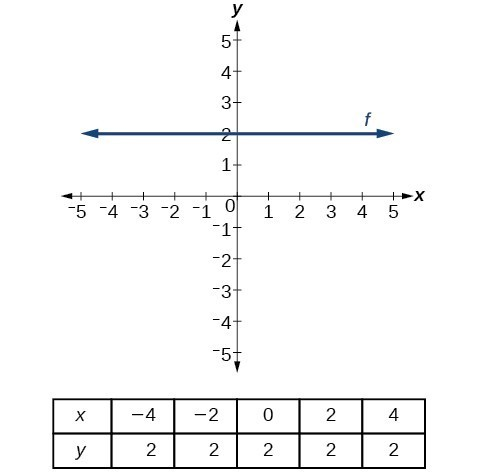A horizontal line representing the function $f\left(x\right)=2$.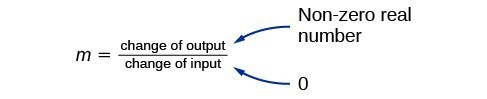A vertical line indicates a constant input or x-value. We can see that the input value for every point on the line is 2, but the output value varies. Because this input value is mapped to more than one output value, a vertical line does not represent a function. Notice that between any two points, the change in the input values is zero. In the slope formula, the denominator will be zero, so the slope of a vertical line is undefined.

Notice that a vertical line has an x-intercept but no y-intercept unless it’s the line x = 0. This graph represents the line x = 2.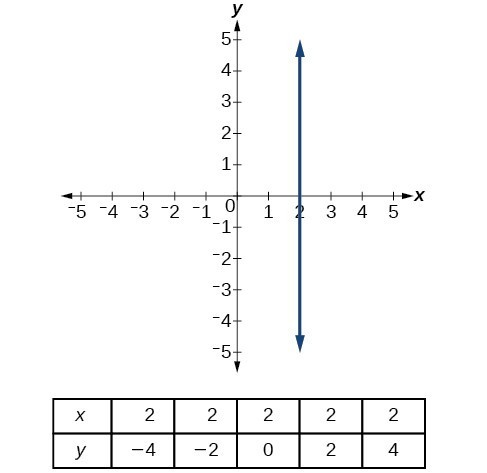The vertical line $x=2$ which does not represent a function.

### A General Note: Horizontal and Vertical Lines

Lines can be horizontal or vertical.

A horizontal line is a line defined by an equation of the form $f\left(x\right)=b$ where $b$ is a constant.

A vertical line is a line defined by an equation of the form $x=a$ where $a$ is a constant.

### Example: Writing the Equation of a Horizontal Line

Write the equation of the line graphed below.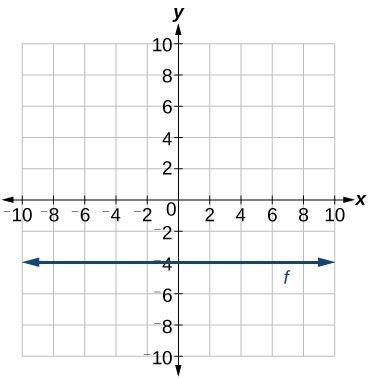### Example: Writing the Equation of a Vertical Line

Write the equation of the line graphed below.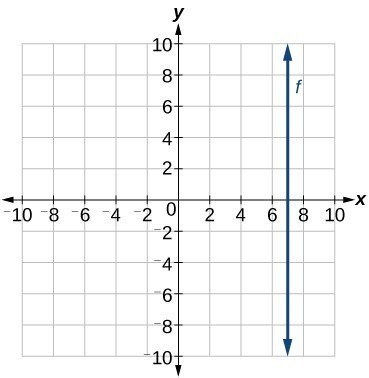### Try It

• Write the equation of the function passing through the points $(2,6)$ and $(4,4)$ in slope-intercept form.
• Write the equation of a function whose slope is 2 and passes through the point $(-1,0)$
• Write the equation of a function whose slope is undefined.

## Parallel and Perpendicular Lines

The two lines in the graph below are parallel lines: they will never intersect. Notice that they have exactly the same steepness which means their slopes are identical. The only difference between the two lines is the y-intercept. If we shifted one line vertically toward the y-intercept of the other, they would become the same line.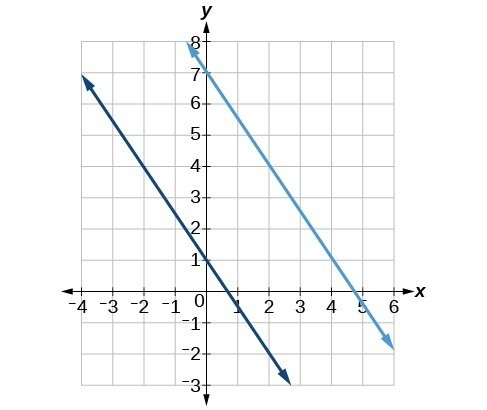Parallel lines.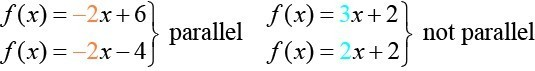We can determine from their equations whether two lines are parallel by comparing their slopes. If the slopes are the same and the y-intercepts are different, the lines are parallel. If the slopes are different, the lines are not parallel.

Unlike parallel lines, perpendicular lines do intersect. Their intersection forms a right or 90-degree angle. The two lines below are perpendicular.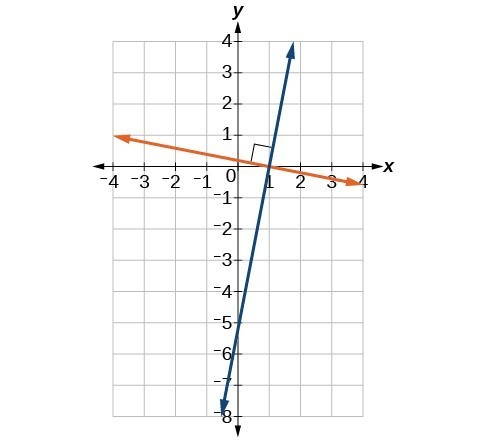Perpendicular lines.

Perpendicular lines do not have the same slope. The slopes of perpendicular lines are different from one another in a specific way. The slope of one line is the negative reciprocal of the slope of the other line. The product of a number and its reciprocal is 1. If ${m}_{1}\text{ and }{m}_{2}$ are negative reciprocals of one another, they can be multiplied together to yield $-1$.

${m}_{1}*{m}_{2}=-1$

To find the reciprocal of a number, divide 1 by the number. So the reciprocal of 8 is $\frac{1}{8}$, and the reciprocal of $\frac{1}{8}$ is 8. To find the negative reciprocal, first find the reciprocal and then change the sign.

As with parallel lines, we can determine whether two lines are perpendicular by comparing their slopes. The slope of each line below is the negative reciprocal of the other so the lines are perpendicular.

$\begin{array}{ll}f\left(x\right)=\frac{1}{4}x+2\hfill & \text{negative reciprocal of }\frac{1}{4}\text{ is }-4\hfill \\ f\left(x\right)=-4x+3\hfill & \text{negative reciprocal of }-4\text{ is }\frac{1}{4}\hfill \end{array}$

The product of the slopes is –1.

$-4\left(\frac{1}{4}\right)=-1$

### A General Note: Parallel and Perpendicular Lines

Two lines are parallel lines if they do not intersect. The slopes of the lines are the same.

$f\left(x\right)={m}_{1}x+{b}_{1}\text{ and }g\left(x\right)={m}_{2}x+{b}_{2}\text{ are parallel if }{m}_{1}={m}_{2}$.

If and only if ${b}_{1}={b}_{2}$ and ${m}_{1}={m}_{2}$, we say the lines coincide. Coincident lines are the same line.

Two lines are perpendicular lines if they intersect at right angles.

$f\left(x\right)={m}_{1}x+{b}_{1}\text{ and }g\left(x\right)={m}_{2}x+{b}_{2}\text{ are perpendicular if }{m}_{1}*{m}_{2}=-1,\text{ and }{m}_{2}=-\frac{1}{{m}_{1}}$.

### Example: Identifying Parallel and Perpendicular Lines

Given the functions below, identify the functions whose graphs are a pair of parallel lines and a pair of perpendicular lines.

$\begin{array}{l}f\left(x\right)=2x+3\hfill & \hfill & h\left(x\right)=-2x+2\hfill \\ g\left(x\right)=\frac{1}{2}x - 4\hfill & \hfill & j\left(x\right)=2x - 6\hfill \end{array}$

### Writing Equations of Parallel Lines

If we know the equation of a line, we can use what we know about slope to write the equation of a line that is either parallel or perpendicular to the given line.

Suppose we are given the following function:

$f\left(x\right)=3x+1$

We know that the slope of the line is 3. We also know that the y-intercept is (0, 1). Any other line with a slope of 3 will be parallel to f(x). The lines formed by all of the following functions will be parallel to f(x).

$\begin{array}{l}g\left(x\right)=3x+6\hfill \\ h\left(x\right)=3x+1\hfill \\ p\left(x\right)=3x+\frac{2}{3}\hfill \end{array}$

Suppose then we want to write the equation of a line that is parallel to f and passes through the point (1, 7). We already know that the slope is 3. We just need to determine which value for b will give the correct line. We can begin by using point-slope form of an equation for a line. We can then rewrite it in slope-intercept form.

$\begin{array}{l}y-{y}_{1}=m\left(x-{x}_{1}\right)\hfill \\ y - 7=3\left(x - 1\right)\hfill \\ y - 7=3x - 3\hfill \\ \text{}y=3x+4\hfill \end{array}$

So $g\left(x\right)=3x+4$ is parallel to $f\left(x\right)=3x+1$ and passes through the point (1, 7).

### How To: Given the equation of a linear function, write the equation of a line WHICH passes through a given point and is parallel to the given line.

1. Find the slope of the function.
2. Substitute the slope and given point into point-slope or slope-intercept form.
3. Simplify.

### Example: Finding a Line Parallel to a Given Line

Find a line parallel to the graph of $f\left(x\right)=3x+6$ that passes through the point (3, 0).

### Writing Equations of Perpendicular Lines

We can use a very similar process to write the equation of a line perpendicular to a given line. Instead of using the same slope, however, we use the negative reciprocal of the given slope. Suppose we are given the following function:

$f\left(x\right)=2x+4$

The slope of the line is 2, and its negative reciprocal is $-\frac{1}{2}$. Any function with a slope of $-\frac{1}{2}$ will be perpendicular to f(x). The lines formed by all of the following functions will be perpendicular to f(x).

$\begin{array}{l}g\left(x\right)=-\frac{1}{2}x+4\hfill \\ h\left(x\right)=-\frac{1}{2}x+2\hfill \\ p\left(x\right)=-\frac{1}{2}x-\frac{1}{2}\hfill \end{array}$

As before, we can narrow down our choices for a particular perpendicular line if we know that it passes through a given point. Suppose that we want to write the equation of a line that is perpendicular to f(x) and passes through the point (4, 0). We already know that the slope is $-\frac{1}{2}$. Now we can use the point to find the y-intercept by substituting the given values into the slope-intercept form of a line and solving for b.

$\begin{array}{l}g\left(x\right)=mx+b\hfill \\ 0=-\frac{1}{2}\left(4\right)+b\hfill \\ 0=-2+b\hfill \\ 2=b\hfill \\ b=2\hfill \end{array}$

The equation for the function with a slope of $-\frac{1}{2}$ and a y-intercept of 2 is

$g\left(x\right)=-\frac{1}{2}x+2$.

So $g\left(x\right)=-\frac{1}{2}x+2$ is perpendicular to $f\left(x\right)=2x+4$ and passes through the point (4, 0). Be aware that perpendicular lines may not look obviously perpendicular on a graphing calculator unless we use the square zoom feature.

### Q & A

A horizontal line has a slope of zero and a vertical line has an undefined slope. These two lines are perpendicular, but the product of their slopes is not –1. Doesn’t this fact contradict the definition of perpendicular lines?

No. For two perpendicular linear functions, the product of their slopes is –1. However, a vertical line is not a function so the definition is not contradicted.

### How To: Given the equation of a linear function, write the equation of a line WHICH passes through a given point and is Perpendicular to the given line.

1. Find the slope of the given function.
2. Determine the negative reciprocal of the slope.
3. Substitute the new slope and the values for x and y from given point into $g\left(x\right)=mx+b$.
4. Solve for b.
5. Write the equation of the line.

### Example: Finding the Equation of a Perpendicular Line

Find the equation of a line perpendicular to $f\left(x\right)=3x+3$ that passes through the point (3, 0).

### Try It

1. For what y-intercept will the graph of$f(x)$ pass through the point $(-2,5)$?
2. Add a new function that uses the slope m that will create a line that is perpendicular to the function $f(x)=mx-2$.
3. For what y-intercept will the new function pass through the point $(4,1)$, and still be perpendicular to $f(x)$

### How To: Given two points on a line and a third point, write the equation of the perpendicular line that passes through the point.

1. Determine the slope of the line passing through the points.
2. Find the negative reciprocal of the slope.
3. Use slope-intercept form or point-slope form to write the equation by substituting the known values.
4. Simplify.

### Example: Finding the Equation of a Line going through a point and Perpendicular to a Given Line

A line passes through the points (–2, 6) and (4, 5). Find the equation of a line that is perpendicular and passes through the point (4, 5).

### Try It

A line passes through the points, (–2, –15) and (2, –3). Find the equation of a perpendicular line that passes through the point, (6, 4).

## Absolute Value FunctionsDistances in deep space can be measured in all directions. As such, it is useful to consider distance in terms of absolute values. (credit: “s58y”/Flickr)

Until the 1920s, the so-called spiral nebulae were believed to be clouds of dust and gas in our own galaxy some tens of thousands of light years away. Then, astronomer Edwin Hubble proved that these objects are galaxies in their own right at distances of millions of light years. Today, astronomers can detect galaxies that are billions of light years away. Distances in the universe can be measured in all directions. As such, it is useful to consider distance as an absolute value function. In this section, we will investigate absolute value functions.

### Understanding Absolute Value

Recall that in its basic form $\displaystyle{f}\left({x}\right)={|x|}$, the absolute value function, is one of our toolkit functions. The absolute value function is commonly thought of as providing the distance a number is from zero on a number line. Algebraically, for whatever the input value is, the output is the value without regard to sign.

### A General Note: Absolute Value Function

The absolute value function can be defined as a piecewise function

$f(x) =\begin{cases}x ,\ x \geq 0 \\ -x , x < 0\\ \end{cases}$

### Example: Determine a Number within a Prescribed Distance

Describe all values $x$ within or including a distance of 4 from the number 5.

### Try It

Describe all values $x$ within a distance of 3 from the number 2.

### Example: Resistance of a Resistor

Electrical parts, such as resistors and capacitors, come with specified values of their operating parameters: resistance, capacitance, etc. However, due to imprecision in manufacturing, the actual values of these parameters vary somewhat from piece to piece even when they are supposed to be the same. The best that manufacturers can do is to try to guarantee that the variations will stay within a specified range, often $\pm 1\%,\pm5\%,$ or $\displaystyle\pm10\%$.

Suppose we have a resistor rated at 680 ohms, $\pm 5\%$. Use the absolute value function to express the range of possible values of the actual resistance.

### Try It

Students who score within 20 points of 80 will pass a test. Write this as a distance from 80 using absolute value notation.

The most significant feature of the absolute value graph is the corner point at which the graph changes direction. This point is shown at the origin.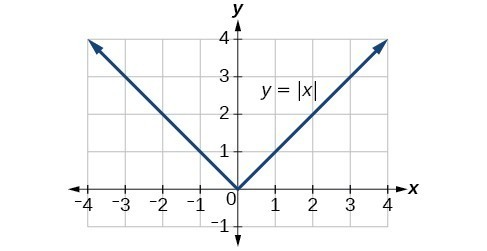The graph below is of $y=2\left|x - 3\right|+4$. The graph of $y=|x|$ has been shifted right 3 units, vertically stretched by a factor of 2, and shifted up 4 units. This means that the corner point is located at $\left(3,4\right)$ for this transformed function.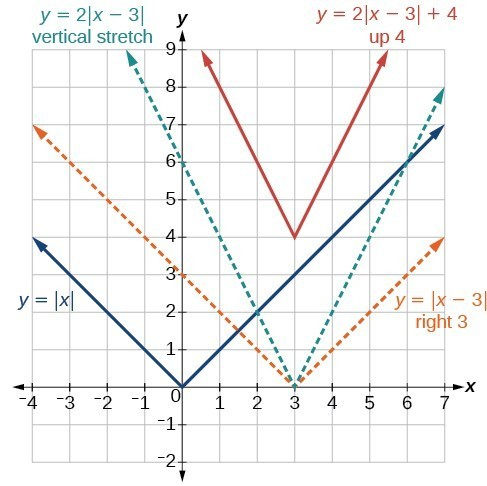### Example: Writing an Equation for an Absolute Value Function

Write an equation for the function graphed below.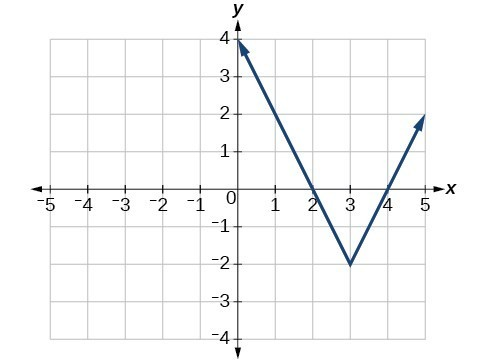Q & A

If we couldn’t observe the stretch of the function from the graphs, could we algebraically determine it?

Yes. If we are unable to determine the stretch based on the width of the graph, we can solve for the stretch factor by putting in a known pair of values for $x$ and $f\left(x\right)$.

$f\left(x\right)=a|x - 3|-2$

Now substituting in the point (1, 2)

$\begin{array}{l}2=a|1 - 3|-2\hfill \\ 4=2a\hfill \\ a=2\hfill \end{array}$

### Try It

Write the equation for the absolute value function that is horizontally shifted left 2 units, vertically flipped, and vertically shifted up 3 units.

Q & A

Do the graphs of absolute value functions always intersect the vertical axis? The horizontal axis?

Yes, they always intersect the vertical axis. The graph of an absolute value function will intersect the vertical axis when the input is zero.

No, they do not always intersect the horizontal axis. The graph may or may not intersect the horizontal axis depending on how the graph has been shifted and reflected. It is possible for the absolute value function to intersect the horizontal axis at zero, one, or two points.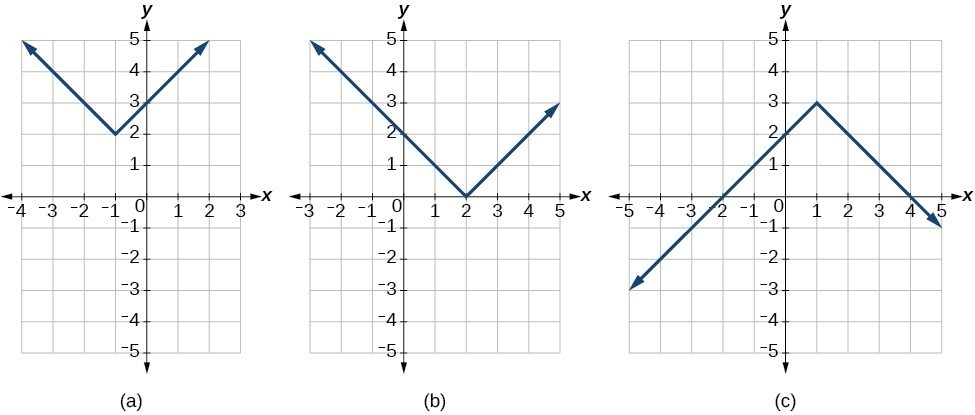(a) The absolute value function does not intersect the horizontal axis. (b) The absolute value function intersects the horizontal axis at one point. (c) The absolute value function intersects the horizontal axis at two points.

### Find the Intercepts of an Absolute Value Function

Knowing how to solve problems involving absolute value functions is useful. For example, we may need to identify numbers or points on a line that are at a specified distance from a given reference point.

### How To: Given the formula for an absolute value function, find the horizontal intercepts of its graph.

1. Isolate the absolute value term.
2. Use $|A|=B$ to write $A=B$ or $\mathrm{-A}=B$, assuming $B>0$.
3. Solve for $x$.

### Example: Finding the Zeros of an Absolute Value Function

For the function $f\left(x\right)=|4x+1|-7$ , find the values of $x$ such that $\text{ }f\left(x\right)=0$ .

### Try It

For the function $f\left(x\right)=|2x - 1|-3$, find the values of $x$ such that $f\left(x\right)=0$.

## Key Concepts

• Linear functions may be graphed by plotting points or by using the y-intercept and slope.
• Graphs of linear functions may be transformed by shifting the graph up, down, left, or right as well as using stretches, compressions, and reflections.
• The y-intercept and slope of a line may be used to write the equation of a line.
• The x-intercept is the point at which the graph of a linear function crosses the x-axis.
• Horizontal lines are written in the form, $f(x)=b$.
• Vertical lines are written in the form, $x=b$.
• Parallel lines have the same slope.
• Perpendicular lines have negative reciprocal slopes, assuming neither is vertical.
• A line parallel to another line, passing through a given point, may be found by substituting the slope value of the line and the x– and y-values of the given point into the equation $f\left(x\right)=mx+b$ and using the b that results. Similarly, point-slope form of an equation can also be used.
• A line perpendicular to another line, passing through a given point, may be found in the same manner, with the exception of using the negative reciprocal slope.
• The absolute value function is commonly used to measure distances between points.
• Applied problems, such as ranges of possible values, can also be solved using the absolute value function.
• The graph of the absolute value function resembles the letter V. It has a corner point at which the graph changes direction.
• In an absolute value equation, an unknown variable is the input of an absolute value function.
• If the absolute value of an expression is set equal to a positive number, expect two solutions for the unknown variable.
• An absolute value equation may have one solution, two solutions, or no solutions.
• An absolute value inequality is similar to an absolute value equation but takes the form $|A|<B,|A|\le B,|A|>B,\text{ or }|A|\ge B$. It can be solved by determining the boundaries of the solution set and then testing which segments are in the set.
• Absolute value inequalities can also be solved graphically.

## Glossary

absolute value equation
an equation of the form $|A|=B$, with $B\ge 0$; it will have solutions when $A=B$ or $-A=B$
absolute value inequality
a relationship in the form $|{ A }|<{ B },|{ A }|\le { B },|{ A }|>{ B },\text{or }|{ A }|\ge{ B }$
horizontal line
a line defined by $f\left(x\right)=b$ where b is a real number. The slope of a horizontal line is 0.
parallel lines
two or more lines with the same slope
perpendicular lines
two lines that intersect at right angles and have slopes that are negative reciprocals of each other
vertical line
a line defined by $x=a$ where a is a real number. The slope of a vertical line is undefined.
x-intercept
the point on the graph of a linear function when the output value is 0; the point at which the graph crosses the horizontal axis